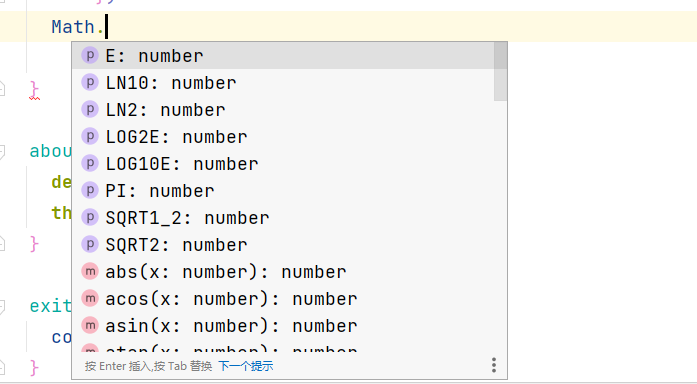# 问一下 ArkTS中math库是哪一个?

math库是哪一个，需要用到，却查不到

HarmonyOS
2023-08-11 15:01:04

/cke_497.png

QQ

2023-08-11 17:40:16

ATS 兼容JavaScript语法

JavaScript的Math库是内置的数学函数库，提供了许多常用的数学操作和函数。下面是一些常见的Math库函数及其用法示例：

1. Math.abs(x): 返回x的绝对值。
``console.log(Math.abs(-5)); // 输出: 5``
1. Math.ceil(x): 返回大于或等于x的最小整数。
``console.log(Math.ceil(4.2)); // 输出: 5``
1. Math.floor(x): 返回小于或等于x的最大整数。
``console.log(Math.floor(4.8)); // 输出: 4``
1. Math.round(x): 返回x的四舍五入值。
``console.log(Math.round(4.5)); // 输出: 5``
1. Math.max(x1, x2, ..., xn): 返回一组数中的最大值。
``console.log(Math.max(2, 5, 1)); // 输出: 5``
1. Math.min(x1, x2, ..., xn): 返回一组数中的最小值。
``console.log(Math.min(2, 5, 1)); // 输出: 1``
1. Math.random(): 返回一个0到1之间的随机数。
``console.log(Math.random()); // 输出: 0.12345（随机数）``
1. Math.sqrt(x): 返回x的平方根。
``console.log(Math.sqrt(16)); // 输出: 4``

QQ

2023-08-12 23:40:214912浏览 • 1回复 待解决

1757浏览 • 1回复 待解决
ob有如何报错，麻烦帮忙看一下
2235浏览 • 1回复 待解决
OpenHarmony UI组件发现了一个好东西!
4494浏览 • 2回复 待解决
entry如何拉起另一个Module的Ability
1558浏览 • 1回复 待解决

6669浏览 • 3回复 已解决

1221浏览 • 1回复 待解决

222浏览 • 1回复 待解决

630浏览 • 1回复 待解决

799浏览 • 5回复 待解决